# Attitude Dynamics of Re-entry Vehicle

Mar. 9, 2012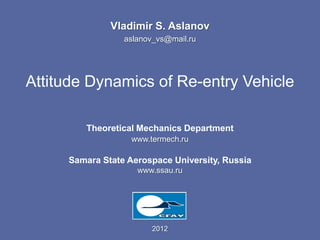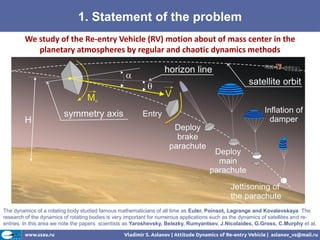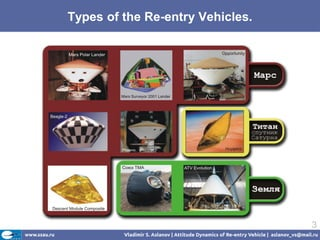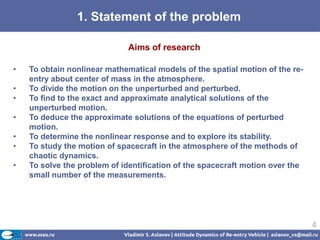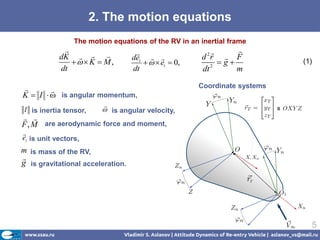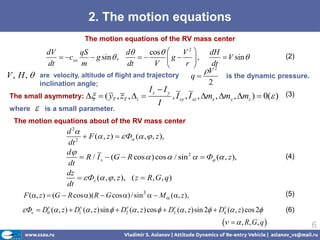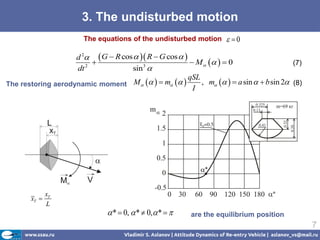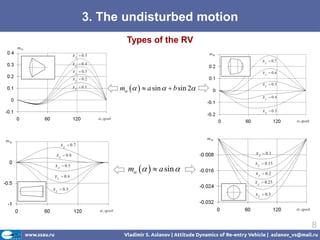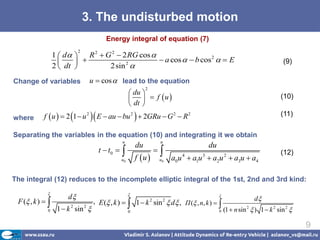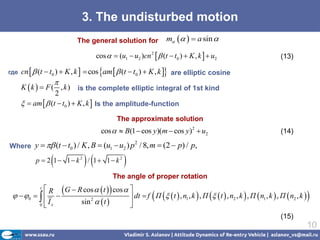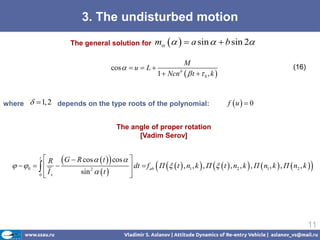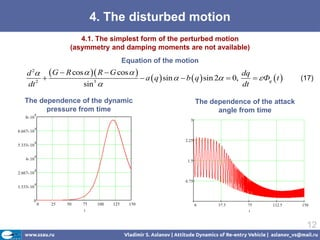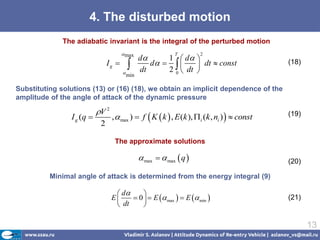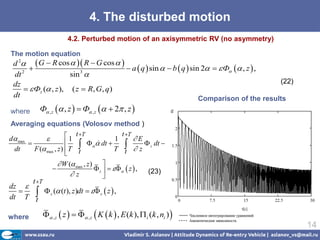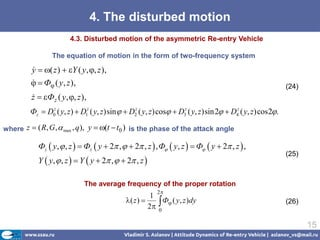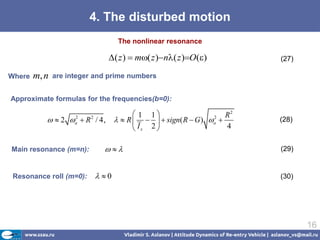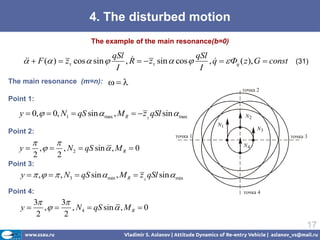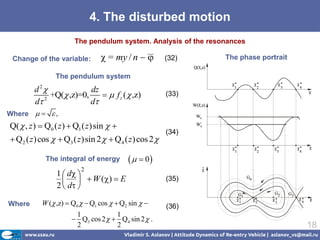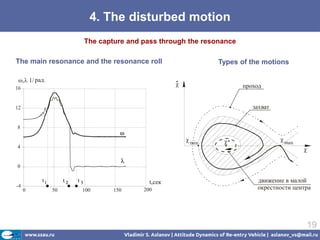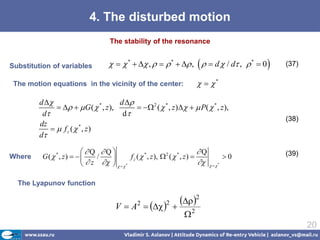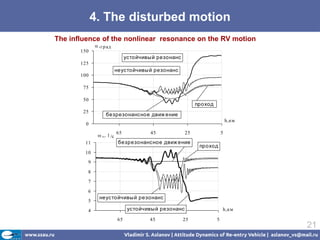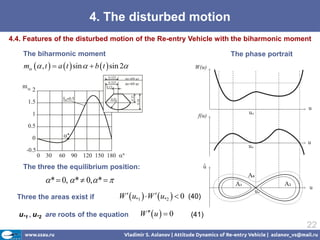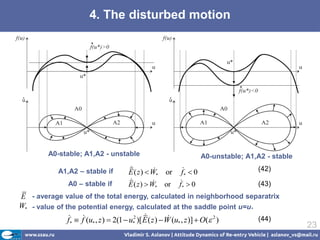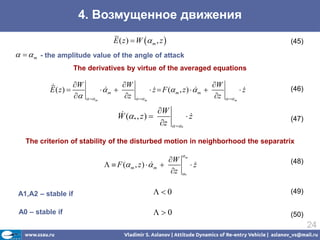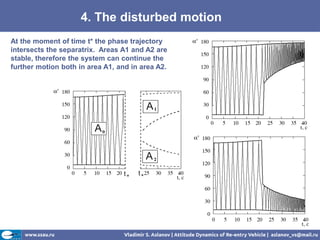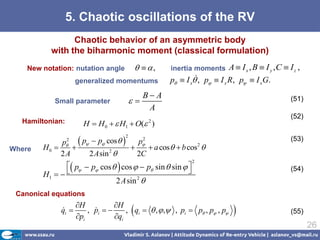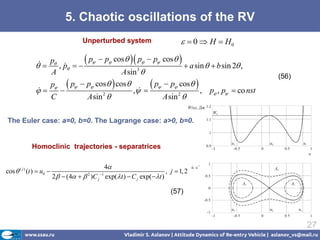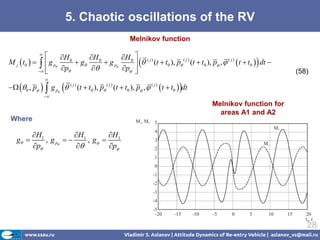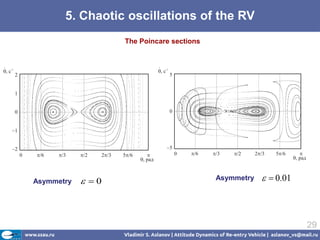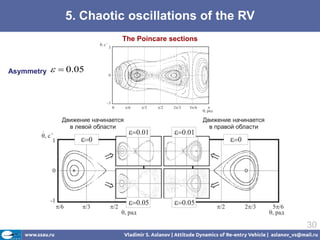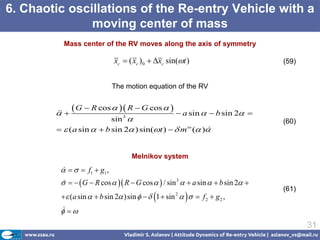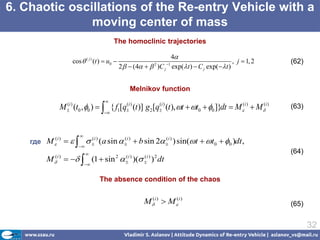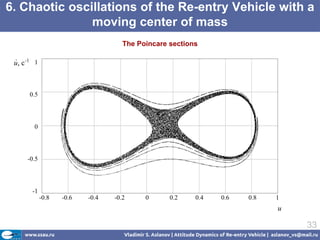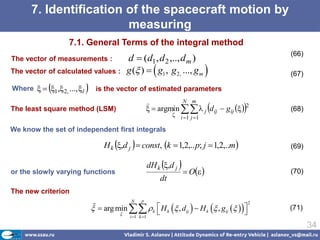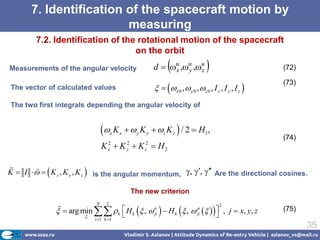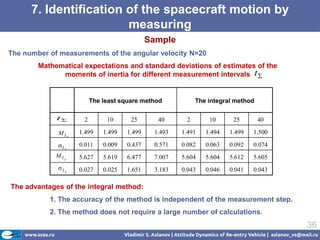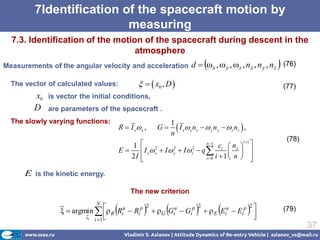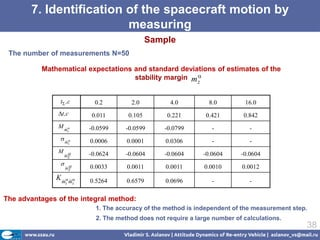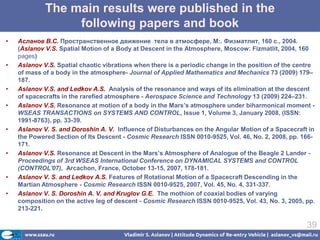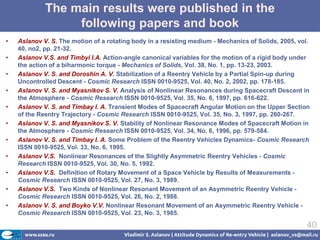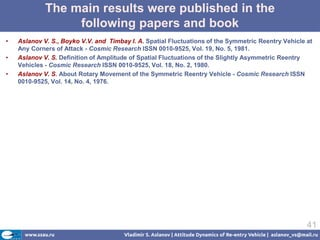1 of 41

### Attitude Dynamics of Re-entry Vehicle

1. Vladimir S. Aslanov aslanov_vs@mail.ru Attitude Dynamics of Re-entry Vehicle Theoretical Mechanics Department www.termech.ru Samara State Aerospace University, Russia www.ssau.ru 2012
2. 1. Statement of the problem We study of the Re-entry Vehicle (RV) motion about of mass center in the planetary atmospheres by regular and chaotic dynamics methods The dynamics of a rotating body studied famous mathematicians of all time as Euler, Poinsot, Lagrange and Kovalevskaya. The research of the dynamics of rotating bodies is very important for numerous applications such as the dynamics of satellites and re- entries. In this area we note the papers scientists as Yaroshevsky, Belezky, Rumyantsev, J.Nicolaides, G.Gross, C.Murphy et al.
3. Types of the Re-entry Vehicles. 3
4. 1. Statement of the problem Aims of research • To obtain nonlinear mathematical models of the spatial motion of the re- entry about center of mass in the atmosphere. • To divide the motion on the unperturbed and perturbed. • To find to the exact and approximate analytical solutions of the unperturbed motion. • To deduce the approximate solutions of the equations of perturbed motion. • To determine the nonlinear response and to explore its stability. • To study the motion of spacecraft in the atmosphere of the methods of chaotic dynamics. • To solve the problem of identification of the spacecraft motion over the small number of the measurements. 4
5. 2. The motion equations The motion equations of the RV in an inertial frame     dK    dei   2 d r  F  K  M,    ei  0, 2 g (1) dt dt dt m  Coordinate systems  K  I   is angular momentum,  I is inertia tensor,  is angular velocity,   F , M are aerodynamic force and moment,  ei is unit vectors, m is mass of the RV,  g is gravitational acceleration. 5
6. 2. The motion equations The motion equations of the RV mass center dV qS d cos   V2  dH  cxa  g sin  ,   g  ,  V sin  (2) dt m dt V  r  dt V 2 V , H,  are velocity, altitude of flight and trajectory q is the dynamic pressure. inclination angle; 2 Iz  I y The small asymmetry:   ( yT , zT , i  , I xy , I xz , mx , my , mz )  0( ) (3) I where  is a small parameter. The motion equations about of the RV mass center d 2 2  F ( , z )   Ф ( ,  , z ), dt d  R / I x  (G  R cos  ) cos  / sin 2   Ф ( , z ), (4) dt dz   Фz ( ,  , z ), ( z  R, G, q ) dt F (, z )  (G  R cos )(R  G cos ) / sin3   M  (, z ), (5)  Ф  D0 ( , z )  D1 ( , z )sin   D ( , z ) cos   D3 ( , z )sin 2  D ( , z) cos 2   2  4 (6)    , R, G, q  6
7. 3. The undisturbed motion The equations of the undisturbed motion  0 d 2  G  R cos   R  G cos     M    0 (7) dt 2 sin 3  qSL The restoring aerodynamic moment M     m   , m    a sin   b sin 2 (8) I xT xT  L  *  0,  *  0, *   are the equilibrium position 7
8. 3. The undisturbed motion Types of the RV m    a sin   b sin 2 m    a sin  8
9. 3. The undisturbed motion Energy integral of equation (7) 1  d  R 2  G 2  2 RG cos  2     a cos   b cos 2   E (9) 2  dt  2sin 2 Change of variables u  cos  lead to the equation 2  du     f u  (10)  dt  where f  u   2 1  u 2  E  au  bu 2   2GRu  G 2  R 2 (11) Separating the variables in the equation (10) and integrating it we obtain u u du du t  t0   u0 f u    u0 a0u  a1u  a2u  a3u  a4 4 3 2 (12) The integral (12) reduces to the incomplete elliptic integral of the 1st, 2nd and 3rd kind:  d   d F ( , k )    , E ( , k )  1  k sin  d , П ( , n, k )   2 2 0 1  k 2 sin 2  0 0 (1  n sin 2  ) 1  k 2 sin 2  9
10. 3. The undisturbed motion The general solution for m    a sin  cos   (u1  u2 )cn 2   (t  t0 )  K , k   u2 (13) где cn   (t  t0 )  K , k   cos am   (t  t0 )  K , k  are elliptic cosine  K  k   F ( , k ) is the complete elliptic integral of 1st kind 2   am   (t  t0 )  K , k  Is the amplitude-function The approximate solution cos   B(1  cos y)(m  cos y)2  u2 (14) Where y   (t  t0 ) / K , B  (u1  u2 ) p / 8, m  (2  p) / p, 2   p  2 1 1 k 2 / 1 1 k 2  The angle of proper rotation  R  G  R cos   t   cos   t   0     sin 2   t    dt  f П   t  , n1 , k  , П   t  , n2 , k  , П  n1 , k  , П  n2 , k   0    Ix  (15) 10
11. 3. The undisturbed motion The general solution for m    a sin   b sin 2 M cos   u  L  (16) 1  Ncn   t 0, k  where   1, 2 depends on the type roots of the polynomial: f u   0 The angle of proper rotation [Vadim Serov]  R  G  R cos   t   cos   t   0     sin 2   t    dt  f ab П   t  , n1 , k  , П   t  , n2 , k  , П  n1 , k  , П  n2 , k   0    Ix  11
12. 4. The disturbed motion 4.1. The simplest form of the perturbed motion (asymmetry and damping moments are not available) Equation of the motion d 2  G  R cos   R  G cos   dq   a  q  sin   b  q  sin 2  0,   Фq  t  (17) dt 2 sin 3  dt The dependence of the dynamic The dependence of the attack pressure from time angle from time 12
13. 4. The disturbed motion The adiabatic invariant is the integral of the perturbed motion  max d 1  d  T 2 Ig    dt d     dt  const 2 0  dt  (18) min Substituting solutions (13) or (16) (18), we obtain an implicit dependence of the amplitude of the angle of attack of the dynamic pressure V 2 I g (q  ,  max )  f  K  k  , E (k ), i (k , ni )   const (19) 2 The approximate solutions  max   max  q  (20) Minimal angle of attack is determined from the energy integral (9)  d  E  0   E  max   E  min  (21)  dt  13
14. 4. The disturbed motion 4.2. Perturbed motion of an axisymmetric RV (no asymmetry) The motion equation d 2  G  R cos   R  G cos     a  q  sin   b  q  sin 2   Ф  , z  , dt 2 sin  3 dz (22)   Фz ( , z ), ( z  R, G, q) dt Comparison of the results where Ф , z  , z   Ф , z   2 , z   Averaging equations (Volosov method ) d max   1 t T 1 t T E F ( max , z )  T  T  z     dt    z dt  dt  t t  W ( max , z )    z     z  , (23) z  t T dz   dt T t   z ( (t ), z)dt   z  z  , where  , z  z    , z  K  k  , E (k ),  i (k , ni )  14
15. 4. The disturbed motion 4.3. Disturbed motion of the asymmetric Re-entry Vehicle The equation of motion in the form of two-frequency system y  ( z )  Y ( y, , z ),    Ф ( y, z ),  (24) z  Фz ( y, , z ),  Фz  D0z ( y, z)  D1z ( y, z) sin  D2z ( y, z) cos  D3z ( y, z) sin 2  D4z ( y, z) cos 2. where z  ( R, G ,  max , q ), y  (t  t0 ) is the phase of the attack angle Фz  y,  , z   Фz  y  2 ,   2 , z  , Ф  y, z   Ф  y  2 , z  , (25) Y  y,  , z   Y  y  2 ,   2 , z  The average frequency of the proper rotation 2 1 2  ( z )  Ф ( y, z )dy (26) 0 15
16. 4. The disturbed motion The nonlinear resonance ( z )  m( z )n( z )O() (27) Where m, n are integer and prime numbers Approximate formulas for the frequencies(b=0):  1 1 R2   2   R / 4, 2 a 2   R     sign( R  G ) a  2 (28)  Ix 2  4 Main resonance (m=n):   (29) Resonance roll (m=0):  0 (30) 16
17. 4. The disturbed motion The example of the main resonance(b=0) qSl  qSl   F ( )  zт cos  sin   , R   zт sin  cos  , q   Фq ( z ), G  const (31)  I I The main resonance (m=n):  точка 2 Point 1: y  0,   0, N1  qS sin  max , M R   z  qSl sin  max N2 N1 N3 Point 2: точка 1 точка 3   y ,  , N 2  qS sin  , M R  0 N4 2 2 Point 3: y   ,    , N3  qS sin  min , M R  z  qSl sin  min Point 4: точка 4 3 3 y ,  , N 4  qS sin  , M R  0 2 2 17
18. 4. The disturbed motion The pendulum system. Analysis of the resonances Change of the variable:  = my / n   (32) The phase portrait The pendulum system d 2 dz +Q( ,z)=0,   f z (  ,z) (33) d 2 d Where    , Q(  , z )  Q0 ( z )  Q1 ( z )sin   (34)  Q2 ( z ) cos   Q3 ( z )sin 2   Q4 ( z ) cos 2  The integral of energy    0 2 1  d     W ()  E (35) 2  d  Where W ( ,z)  Q0   Q1 cos   Q2 sin   (36) 1 1  Q3 cos 2   Q4 sin 2  . 2 2 18
19. 4. The disturbed motion The capture and pass through the resonance The main resonance and the resonance roll Types of the motions  рад.  проход захват  min *  max  t,сек движение в малой 0 50 100 150 200 окрестности центра 19
20. 4. The disturbed motion The stability of the resonance Substitution of variables    *   ,    *   ,    d  / d ,  *  0  (37) The motion equations in the vicinity of the center:   * d  d      G (  * , z ),   2 (  * , z )   P(  * , z ), d d (38) dz   f z ( * , z) d Q Q Q (39) G( * , z)    f z (  * , z ),  2 (  * , z )  0  z      * Wherе /       * The Lyapunov function VA 2     2 2 2  20
21. 4. The disturbed motion The influence of the nonlinear resonance on the RV motion   гр ад 150 устойчивы й резонанс 125 неустойчивы й резонанс 100 75 50 проход 25 безрезонансное движ ение h,км 0 65 45 25 5  к  /с 11 безрезонансное движ ение проход 10 9 8 7 6 неустойчивы й резонанс 5 4 устойчивы й резонанс h,км 65 45 25 5 21
22. 4. The disturbed motion 4.4. Features of the disturbed motion of the Re-entry Vehicle with the biharmonic moment The biharmonic moment The phase portrait m  , t   a  t  sin   b  t  sin 2 The three the equilibrium position:  *  0,  *  0, *   Three the areas exist if W   u*1   W   u*2   0 (40) u*1 , u*2 are roots of the equation W   u   0 (41) 22
23. 4. The disturbed motion A0-stable; A1,A2 - unstable A0-unstable; A1,A2 - stable A1,A2 – stable if    E ( z )  W* or f*  0 (42) A0 – stable if    E ( z )  W* or f*  0 (43) E - average value of the total energy, calculated in neighborhood separatrix W* - value of the potential energy, calculated at the saddle point u=u*     f  f (u , z )  2(1  u 2 )[ E ( z )  W (u , z )]  O( 2 ) (44) * * * * 23
24. 4. Возмущенное движения E ( z )  W  m , z  (45)    m - the amplitude value of the angle of attack The derivatives by virtue of the averaged equations  W W W E ( z)  m    z  F ( m , z )   m    z  (46)    m z   m z   m  W W (* , z )  z  (47) z  * The criterion of stability of the disturbed motion in neighborhood the separatrix m W (48)   F ( m , z )   m   z  z * A1,A2 – stable if 0 (49) A0 – stable if 0 (50) 24
25. 4. The disturbed motion At the moment of time t* the phase trajectory intersects the separatrix. Areas A1 and A2 are stable, therefore the system can continue the further motion both in area A1, and in area A2.
26. 5. Chaotic oscillations of the RV Chaotic behavior of an asymmetric body with the biharmonic moment (classical formulation) New notation: nutation angle   , inertia moments A  I x , B  I y , C  I z , generalized momentums  p  I x , p  I x R, p  I xG. B A Small parameter  (51) A (52) Hamiltonian: H  H 0   H1  O( ) 2  p  p cos  2 2 2 p (53) p Where H0      a cos   b cos  2 2A 2 A sin 2  2C  p  p cos   cos   p sin  sin   2 H1     (54) 2 A sin 2  Canonical equations H H qi   pi , pi    qi  , qi   ,  , , pi  p , p , p  (55) 26
27. 5. Chaotic oscillations of the RV Unperturbed system   0  H  H0   p , p     p  p cos   p  p cos      a sin   b sin 2 , A A sin 3  (56) p  p  p cos   cos   p  p cos  , p , p  co nst    ,    C A sin 2  A sin 2  The Euler case: a=0, b=0. The Lagrange case: a>0, b=0. Homoclinic trajectories - separatrices 4 cos  ( j ) (t )  u0  , j  1, 2 2   (4   )C j exp(t )  C j exp( t ) 2 1 (57) 27
28. 5. Chaotic oscillations of the RV Melnikov function   H 0 H 0 H 0  ( j ) M j  t0     g p  g  g p   (t  t0 ), p (t  t0 ), p ,   t  t0   dt  ( j) ( j)    p  p  (58)    0 , p   g p  ( j ) (t  t0 ), p ( j ) (t  t0 ), p ,  ( j )  t  t0  dt  Melnikov function for areas А1 and А2 Where H1 H H1 g  , g p   1 , g  p  p 28
29. 5. Chaotic oscillations of the RV The Poincare sections Asymmetry  0 Asymmetry   0.01 29
30. 5. Chaotic oscillations of the RV The Poincare sections Asymmetry   0.05 30
31. 6. Chaotic oscillations of the Re-entry Vehicle with a moving center of mass Mass center of the RV moves along the axis of symmetry xc  ( xc )0  xc sin(t ) (59) The motion equation of the RV    G  R cos   R  G cos    a sin   b sin 2  sin  3 (60)   ( a sin   b sin 2 ) sin(t )   m ( ) Melnikov system     f1  g1 ,      G  R cos   R  G cos   / sin 3   a sin   b sin 2   (61)  (a sin   b sin 2 ) sin    1  sin     f 2  g 2 , 2    31
32. 6. Chaotic oscillations of the Re-entry Vehicle with a moving center of mass The homoclinic trajectories 4 cos  ( j ) (t )  u0  , j  1, 2 (62) 2   (4   )C j exp(t )  C j exp( t ) 2 1 Melnikov function  M (t0 , 0 )   { f1[qi ) (t )] g2[qi ) (t ), t  t0  0 ]}dt  M (i )  M (i ) (i )  ( ( (63)   где M      i ) (a sin  i )  b sin 2 i ) ) sin(t  t0  0 )dt , (i ) ( ( (   (64) M     (1  sin  )( ) dt (i ) 2 (i )  (i ) 2   The absence condition of the chaos M(i )  M (i ) (65) 32
33. 6. Chaotic oscillations of the Re-entry Vehicle with a moving center of mass The Poincare sections 33
34. 7. Identification of the spacecraft motion by measuring 7.1. General Terms of the integral method (66) The vector of measurements : d  (d1 , d 2 ,.., d m ) The vector of calculated values : g ( )   g1 , g 2, ..., g m  (67)  Where   1 , 2, ..., l  is the vector of estimated parameters    N m The least square method (LSM)   argmin   j d ij  g ij   2 (68)  i 1 j 1 We know the set of independent first integrals   H k ,d j  const, k  1,2,.. p; j  1,2,..m (69) or the slowly varying functions  dH k ,d j   O (70) dt The new criterion  k  H k  , dij   H k  , gij    N p 2    arg min (71)  i 1 k 1   34
35. 7. Identification of the spacecraft motion by measuring 7.2. Identification of the rotational motion of the spacecraft on the orbit Measurements of the angular velocity x d  u ,u ,u y z  (72)    x 0 ,  y 0 ,  z 0 , I x , I y , I z  (73) The vector of calculated values The two first integrals depending the angular velocity of  K x x   y K y   z K z  / 2  H1 , (74) K  K  K  H2 2 x 2 y 2 z  K  I     K x , K y , K z  is the angular momentum, , ,  Are the directional cosines.  The new criterion  k  H k  ,  uji   H k  ,  jip    , j  x, y, z N 2 2    arg min (75)   i 1 k 1  35
36. 7. Identification of the spacecraft motion by measuring Sample The number of measurements of the angular velocity N=20 Mathematical expectations and standard deviations of estimates of the moments of inertia for different measurement intervals t  The least square method The integral method t 2 10 25 40 2 10 25 40 MIx 1.499 1.499 1.499 1.493 1.491 1.494 1.499 1.500 I x 0.011 0.009 0.437 0.571 0.082 0.063 0.092 0.074 MIy 5.627 5.619 6.477 7.007 5.604 5.604 5.612 5.605 I y 0.027 0.025 1.651 3.183 0.043 0.046 0.041 0.043 The advantages of the integral method: 1. The accuracy of the method is independent of the measurement step. 2. The method does not require a large number of calculations. 36
37. 7Identification of the spacecraft motion by measuring 7.3. Identification of the motion of the spacecraft during descent in the atmosphere  Measurements of the angular velocity and acceleration d  x ,  y , z , nx , n y , nz (76)  The vector of calculated values:    x0 , D  (77) x0 is vector the initial conditions, D are parameters of the spacecraft . The slowly varying functions: R  I x x , G 1 n  I x x nx   y n y   z nz  , (78) 1  ci  nx   n 1 i 1 E  I x x  I  y  I  z  q     2 2 2 2I   i 0 i  1  n    E is the kinetic energy. The new criterion       N   argmin   R Riu  Rip   E Eiu  Eip  ~ 2 2 2  G Giu  Gip (79)  i 1     37
38. 7. Identification of the spacecraft motion by measuring Sample The number of measurements N=50 Mathematical expectations and standard deviations of estimates of the stability margin m  z t ,c 0.2 2.0 4.0 8.0 16.0 t ,c 0.011 0.105 0.221 0.421 0.842 M m  -0.0599 -0.0599 -0.0799 - - z  m  0.0006 0.0001 0.0306 - - z M ~ -0.0624 -0.0604 -0.0604 -0.0604 -0.0604 mz  ~ 0.0033 0.0011 0.0011 0.0010 0.0012 mz K m m  ~ 0.5264 0.6579 0.0696 - - z z The advantages of the integral method: 1. The accuracy of the method is independent of the measurement step. 2. The method does not require a large number of calculations. 38
39. The main results were published in the following papers and book • Асланов В.С. Пространственное движение тела в атмосфере, М:. Физматлит, 160 с., 2004. (Aslanov V.S. Spatial Motion of a Body at Descent in the Atmosphere, Moscow: Fizmatlit, 2004, 160 pages) • Aslanov V.S. Spatial chaotic vibrations when there is a periodic change in the position of the centre of mass of a body in the atmosphere- Journal of Applied Mathematics and Mechanics 73 (2009) 179– 187. • Aslanov V.S. and Ledkov A.S. Analysis of the resonance and ways of its elimination at the descent of spacecrafts in the rarefied atmosphere - Aerospace Science and Technology 13 (2009) 224–231. • Aslanov V.S. Resonance at motion of a body in the Mars’s atmosphere under biharmonical moment - WSEAS TRANSACTIONS on SYSTEMS AND CONTROL, Issue 1, Volume 3, January 2008, (ISSN: 1991-8763), pp. 33-39. • Aslanov V. S. and Doroshin A. V. Influence of Disturbances on the Angular Motion of a Spacecraft in the Powered Section of Its Descent - Cosmic Research ISSN 0010-9525, Vol. 46, No. 2, 2008, pp. 166- 171. • Aslanov V.S. Resonance at Descent in the Mars’s Atmosphere of Analogue of the Beagle 2 Lander - Proceedings of 3rd WSEAS International Conference on DYNAMICAL SYSTEMS and CONTROL (CONTROL'07), Arcachon, France, October 13-15, 2007, 178-181. • Aslanov V. S. and Ledkov A.S. Features of Rotational Motion of a Spacecraft Descending in the Martian Atmosphere - Cosmic Research ISSN 0010-9525, 2007, Vol. 45, No. 4, 331-337. • Aslanov V. S. Doroshin A. V. and Kruglov G.E. The mothion of coaxial bodies of varying composition on the active leg of descent - Cosmic Research ISSN 0010-9525, Vol. 43, No. 3, 2005, pp. 213-221. 39
40. The main results were published in the following papers and book • Aslanov V. S. The motion of a rotating body in a resisting medium - Mechanics of Solids, 2005, vol. 40, no2, pp. 21-32. • Aslanov V.S. and Timbyi I.A. Action-angle canonical variables for the motion of a rigid body under the action of a biharmonic torque - Mechanics of Solids, Vol. 38, No. 1, pp. 13-23, 2003. • Aslanov V. S. and Doroshin A. V. Stabilization of a Reentry Vehicle by a Partial Spin-up during Uncontrolled Descent - Cosmic Research ISSN 0010-9525, Vol. 40, No. 2, 2002, pp. 178-185. • Aslanov V. S. and Myasnikov S. V. Analysis of Nonlinear Resonances during Spacecraft Descent in the Atmosphere - Cosmic Research ISSN 0010-9525, Vol. 35, No. 6, 1997, pp. 616-622. • Aslanov V. S. and Timbay I. A. Transient Modes of Spacecraft Angular Motion on the Upper Section of the Reentry Trajectory - Cosmic Research ISSN 0010-9525, Vol. 35, No. 3, 1997, pp. 260-267. • Aslanov V. S. and Myasnikov S. V. Stability of Nonlinear Resonance Modes of Spacecraft Motion in the Atmosphere - Cosmic Research ISSN 0010-9525, Vol. 34, No. 6, 1996, pp. 579-584. • Aslanov V. S. and Timbay I. A. Some Problem of the Reentry Vehicles Dynamics- Cosmic Research ISSN 0010-9525, Vol. 33, No. 6, 1995. • Aslanov V.S. Nonlinear Resonances of the Slightly Asymmetric Reentry Vehicles - Cosmic Research ISSN 0010-9525, Vol. 30, No. 5, 1992. • Aslanov V.S. Definition of Rotary Movement of a Space Vehicle by Results of Measurements - Cosmic Research ISSN 0010-9525, Vol. 27, No. 3, 1989. • Aslanov V.S. Two Kinds of Nonlinear Resonant Movement of an Asymmetric Reentry Vehicle - Cosmic Research ISSN 0010-9525, Vol. 26, No. 2, 1988. • Aslanov V. S. and Boyko V.V. Nonlinear Resonant Movement of an Asymmetric Reentry Vehicle - Cosmic Research ISSN 0010-9525, Vol. 23, No. 3, 1985. 40
41. The main results were published in the following papers and book • Aslanov V. S., Boyko V.V. and Timbay I. A. Spatial Fluctuations of the Symmetric Reentry Vehicle at Any Corners of Attack - Cosmic Research ISSN 0010-9525, Vol. 19, No. 5, 1981. • Aslanov V. S. Definition of Amplitude of Spatial Fluctuations of the Slightly Asymmetric Reentry Vehicles - Cosmic Research ISSN 0010-9525, Vol. 18, No. 2, 1980. • Aslanov V. S. About Rotary Movement of the Symmetric Reentry Vehicle - Cosmic Research ISSN 0010-9525, Vol. 14, No. 4, 1976. 41